# 数字控制的CCM Boost PFC变换器的实现方法Realization of CCM Boost PFC Converter Based on Digital Control

• 全文下载: PDF(1011KB)    PP.199-205   DOI: 10.12677/JEE.2018.62023
• 下载量: 601  浏览量: 1,726

Based on the basic principle of average current control, the duty cycle expression of the control algorithm is derived, and a digital realization method of variable duty cycle control with constant power is proposed in this paper. The 300 W CCM Boost PFC converter is experimentally verified based on digital signal controller MC56F8257. The experimental results show that the digital control can achieve a power factor close to 1 in a wide input voltage range, and the distortion of input current is very small.

1. 引言

2. 平均电流控制的原理

2.1. Boost PFC工作模式分析

Boost PFC变换器的主功率电路如图1所示。

Boost PFC变换器工作于电感电流连续导通模式时，在一个开关周期内，电感电流始终不会降至零，一直处于连续状态。CCM模式下的Boost PFC变换器在一个开关周期内的电感电流有两种状态：MOS管导通状态与续流二极管导通状态，等效电路图如图2所示。为了不失一般性的分析，假设此时变换器工作于第n个开关周期内，经整流桥整流后的输入电压为：

${v}_{g}\left(n\right)={V}_{m}|\mathrm{sin}\left(\omega n{T}_{s}\right)|$ (1)Figure 1. Boost PFC converter circuit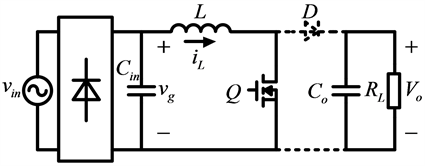(a)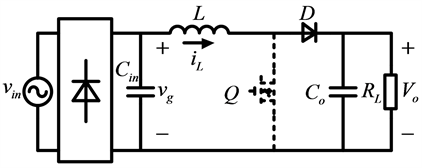(b)

Figure 2. Working mode of CCM Boost PFC converter. (a) Mode 1, (b) Mode 2

${i}_{L}\left(t\right)={i}_{L_initial}\left(n\right)+\frac{{v}_{g}\left(n\right)}{L}t$ (2)

${v}_{L}\left(t\right)=L\frac{\text{d}{i}_{L}\left(t\right)}{\text{d}t}={v}_{g}={V}_{m}|\mathrm{sin}\left(\omega n{T}_{s}\right)|$ (3)

${i}_{L}\left(t\right)={i}_{L_Ton}+\frac{{v}_{g}\left(n\right)-{V}_{o}}{L}t$ (4)

${v}_{L}\left(t\right)=L\frac{\text{d}{i}_{L}}{\text{d}t}={v}_{g}\left(t\right)-{V}_{o}={V}_{m}|\mathrm{sin}\left(\omega n{T}_{s}\right)|-{V}_{o}$ (5)

${\int }_{0}^{{T}_{s}}{v}_{L}\left(t\right)\text{d}t={V}_{m}|\mathrm{sin}\left(\omega n{T}_{s}\right)|{T}_{on}+\left[{V}_{m}|\mathrm{sin}\left(\omega n{T}_{s}\right)|-{V}_{o}\right]{T}_{off}=0$ (6)

$D\left(t\right)=1-\frac{{V}_{m}|\mathrm{sin}\left(\omega n{T}_{s}\right)|}{{V}_{o}}$ (7)

2.2. 控制策略分析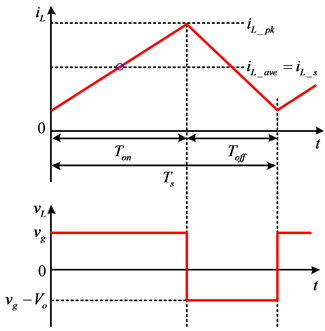Figure 3. Inductor current and voltage waveform of CCM Boost PFC converter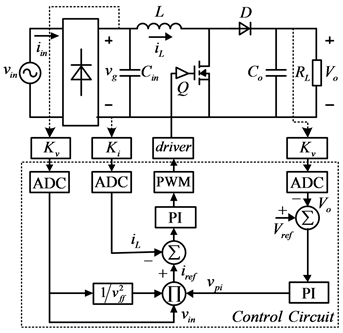Figure 4. Average current digital control principle block diagram

Boost电路组成，控制电路包括采样调理电路、驱动电路和DSP周边电路。

${i}_{ref}=\frac{{V}_{PI}{V}_{in}}{{V}_{ff}^{2}}$ (8)

${V}_{ff}=\frac{1}{\text{π}}{\int }_{0}^{\text{π}}{V}_{m}|\mathrm{sin}\left(\omega t\right)|\text{d}t=\frac{2{V}_{m}}{\text{π}}$ (9)

3. 数字控制的前馈环节

3.1. 输入电压前馈环节

${P}_{in}={I}_{ac_rms}{V}_{ac_rms}$ (10)

${P}_{o}=\eta {P}_{in}$ (11)

APFC变换器的输出功率在工频周期内只和负载有关，当负载不变时，输出功率应该为定值。当输入电压变化为kVac_rms时，由式(8~9)可得参考电流变为

${{i}^{\prime }}_{ref}=\frac{{V}_{PI}k{V}_{ac_rms}}{{k}^{2}{V}_{ff}^{2}}=\frac{{V}_{PI}{V}_{ac_rms}}{k{V}_{ff}^{2}}$ (12)

${{P}^{\prime }}_{in}=k{V}_{ac_rms}×\frac{{I}_{ac_rms}}{k}={P}_{in}$ (13)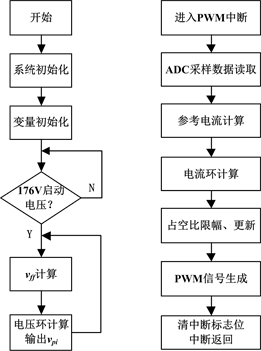Figure 5. Flow chart of control program

3.2. 占空比前馈环节

APFC变换器在输入电压过零点附近存在输入电流畸变现象，电流畸变的主要原因是电感电流的相位超前输入电压造成的。在模拟控制中利用电容和电阻构成相位补偿网络来补偿这个相位超前  ，由于这种方法需要外加电路，并没有得到很好的推广。常见的抑制输入电流畸变的方法是在电流环输出环节加上占空比前馈值，减小输入电压和输出电压对输入电流的影响。这种方法由于程序简单，尤其适合数字控制电路。

$L\frac{\text{d}{\stackrel{^}{i}}_{L}}{\text{d}t}={\stackrel{^}{v}}_{in}-\left(1-D\right){\stackrel{^}{v}}_{o}+\stackrel{^}{d}{V}_{o}$ (14)

$L\frac{\text{d}{\stackrel{^}{i}}_{L}}{\text{d}t}={\stackrel{^}{d}}^{\prime }{V}_{o}$ (15)

$sL{\stackrel{^}{i}}_{L}\left(s\right)={\stackrel{^}{v}}_{in}\left(s\right)-\left(1-D\right){\stackrel{^}{v}}_{o}\left(s\right)+\stackrel{^}{d}\left(s\right){V}_{o}$ (16)

$sL{\stackrel{^}{i}}_{L}\left(s\right)={\stackrel{^}{d}}^{\prime }\left(s\right){V}_{o}$ (17)

$\Delta \stackrel{^}{d}\left(s\right)={\stackrel{^}{d}}^{\prime }\left(s\right)-\stackrel{^}{d}\left(s\right)=\frac{\left(1-D\right){\stackrel{^}{v}}_{o}\left(s\right)-{\stackrel{^}{v}}_{in}\left(s\right)}{{V}_{o}}$ (18)

$sL{\stackrel{^}{i}}_{L}\left(s\right)={\stackrel{^}{v}}_{in}\left(s\right)-\left(1-D\right){\stackrel{^}{v}}_{o}\left(s\right)+\left[\stackrel{^}{d}\left(s\right)+\Delta {\stackrel{^}{d}}^{\prime }\left(s\right)\right]{V}_{o}=\stackrel{^}{d}\left(s\right){V}_{o}$ (19)

$\Delta d=1-\frac{{V}_{in}}{{V}_{o}}$ (20)

4. 实验验证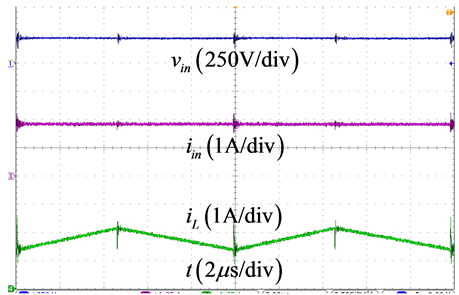(a)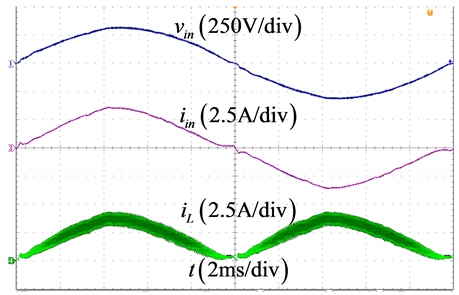(b)

Figure 6. Input voltage, input current and inductor current waveforms. (a) Power frequency cycles, (b) Switching cyclesTable 1. PF and THD at different input voltages

5. 结语

  朱锋, 龚春英. 单周期控制Boost PFC变换器分析与设计[J]. 电力电子技术, 2007, 41(1): 100-102.  陶海燕, 钱承山, 毛鹏, 等. Boost PFC变换器数字控制研究[J]. 电子器件, 2013, 36(6): 889-893.  Jiang, Y.M. (1998) High Effi-ciency Boost Converters for Wide Range Single-Phase AC Input Power Factor Correction. INTELEC, Twentieth Interna-tional Telecommunications Energy Conference (Cat. No.98CH36263), San Francisco, CA, 4-8 October 1998, 603-606.  Chen, F.Z. and Maksimović, D. (2010) Digital Control for Improved Efficiency and Reduced Harmonic Distortion over Wide Load Range in Boost PFC Rectifiers. IEEE Transactions on Power Electronics, 25, 2683-2692.  李玉玲, 吴健儿, 张仲超. 功率因数校正技术的控制策略综述[J]. 通信电源技术, 2003(6): 24-28.  王少永, 张方华. 平均电流型Boost PFC变换器中过零畸变[J]. 电工技术学报, 2011, 26(2): 129-133 + 155.  Sype, D.M.V.D., Gusseme, K.D., Bossche, A.P.M.V.D., et al. (2005) Duty-Ratio Feed Forward for Digitally Controlled Boost PFC Converters. IEEE Transactions on Industrial Electronics, 52, 108-115. https://doi.org/10.1109/TIE.2004.841127